## The Fundamental Theorem of Calculus

Let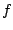be a continuous function on the interval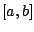. The following theorem is incredibly useful in mathematics, physics, biology, etc.

Theorem 2.1.3   If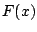is any differentiable function onsuch that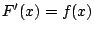, then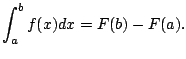One reason this is amazing, is because it says that the area under the entire curve is completely determined by the values of a (magic'') auxiliary function at only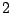points. It's hard to believe. It reduces computing (2.1.2) to finding a single function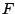, which one can often do algebraically, in practice.Whether or not one should use this theorem to evaluate an integral depends a lot on the application at hand, of course. One can also use a partial limit via a computer for certain applications (numerical integration).

Example 2.1.4   I've always wondered exactly what the area is under a hump'' of the graph of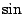. Let's figure it out, using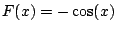.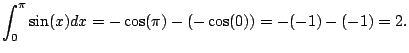But does such analways exist? The surprising answer is yes''.

Theorem 2.1.5   Let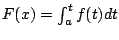. Thenfor all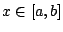.

Note that a nice formula'' forcan be hard to find or even provably non-existent.

The proof of Theorem 2.1.5 is somewhat complicated but is given in complete detail in Stewart's book, and you should definitely read and understand it.

Proof. [Sketch of Proof] We use the definition of derivative.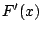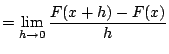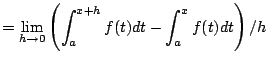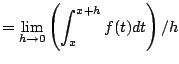Intuitively, for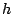sufficiently smallis essentially constant, so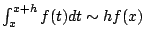(this can be made precise using the extreme value theorem). Thus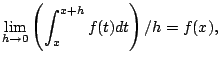which proves the theorem.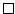William Stein 2006-03-15